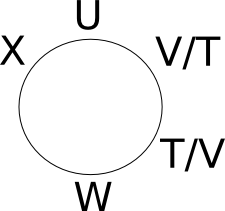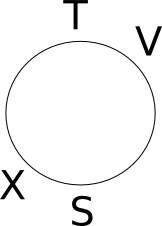0
2582

# Data Sufficiency Questions For IBPS PO PDF:

Download Data Sufficiency Questions For IBPS PO PDF. Practice Data Sufficiency Questions with Solutions for Banking exams based on asked questions in previous papers.

Directions: Each of the questions below consists of a question and two statements numbered I and II given below it. You have to decide whether the data given in the statements are sufficient to answer the questions.

a) The data in statement I alone are sufficient to answer the question, while the data in statement II alone are not sufficient to answer the question.

b) The data in statement II alone are sufficient to answer the question, while the data in statement I alone are not sufficient to answer the question.

c) The data either in statement I alone or statement II alone are sufficient to answer the question.

d) The data in statement I and II together are not sufficient to answer the question.

e) The data in both statements I and II together are necessary to answer the question.

Question 1: Seventeen people are standing in a straight line facing south. What is Bhavna’s position from the left end of the line?

I. Sandeep is standing second to the left of Sheetal. Only five people stand between Sheetal and the one who is standing at the extreme right end of the line. Four people stand between Sandeep and Bhavna.

II. Anita is standing fourth to the left of Sheetal. Less than three people are standing between Bhavna and Anita.

a) The data in statement I alone are sufficient to answer the question, while the data in statement II alone are not sufficient to answer the question.
b) The data in statement II alone are sufficient to answer the question, while the data in statement I alone are not sufficient to answer the question.
c) The data either in statement I alone or statement II alone are sufficient to answer the question.
d) The data in statement I and II together are not sufficient to answer the question.
e)The data in both statements I and II together are necessary to answer the question.

Question 2: Five letters – A, E, G, N and R – are arranged from left to right according to certain conditions. Which letter is placed third?

I. G is placed second to the right of A. E is on the immediate right of G. There are only two letters between R and G.
II. N is exactly between A and G. Neither A nor G is at the extreme and of the arrangement.

a) The data in statement I alone are sufficient to answer the question, while the data in statement II alone are not sufficient to answer the question.
b) The data in statement II alone are sufficient to answer the question, while the data in statement I alone are not sufficient to answer the question.
c) The data either in statement I alone or statement II alone are sufficient to answer the question.
d) The data in statement I and II together are not sufficient to answer the question.
e)The data in both statements I and II together are necessary to answer the question.

Question 3: Six people – S, T, U, V, W and X – are sitting around a circular table facing the centre. What is T’s position with respect to X?

I. Only two people sit between U and W. X is second to the left of W. V and T are immediate neighbours of each other.
II. T is to the immediate right of V. There are only two people between T and S. X is an immediate neighbour of S but not of V.

a) The data in statement I alone are sufficient to answer the question, while the data in statement II alone are not sufficient to answer the question.
b) The data in statement II alone are sufficient to answer the question, while the data in statement I alone are not sufficient to answer the question.
c) The data either in statement I alone or statement II alone are sufficient to answer the question.
d) The data in statement I and II together are not sufficient to answer the question.
e)The data in both statements I and II together are necessary to answer the question.

Instructions: Each of the following questions consists of a question followed by three statements I, II and III. You have to study the question and the statements and decide which of the statement (s) is/are necessary to answer the question.

Question 4: What is the speed of boat in still water?
I. The boat covers 12 km in 2 hours in downstream.
II. The boat covers same distance in 4 hours in upstream.
III. The speed of stream is one third of that of boat in still water.

a) Both I and II
b) I and either II or III
c) All I, II and III
d) Question cannot be answered even with the information in all three statements
e)None of these

Question 5: What is the speed of train?
I. The length of train is 240 metre.
II. The train crosses a pole in 24 seconds.
III. The train crosses a platform in 48 seconds.

a) Both I and III
b) Both I and II
c) Both II and III
d) Any two of three
e) None of these

Question 6: What is the age of class teacher?
I. There are 11 students in the class.
II. The average age of students and the teacher is 14 years.
III. The average age of the teacher and students is 3 years more than that of students.

a) Both I and III
b) Both I and II
c) II and either I or III
d) All I, II and III
e) None of these

Question 7: Sri Gupta borrowed a sum at compound interest. What is the amount returned in 2 years?
I. The rate of interest is 5% per annum.
II. The simple interest incurred on the sum in 1 year is Rs600.
III. The borrowed sum is ten times the amount earned as simple interest in two years.

a) Only I
b) Only III
c) Both II and III
d) Either I or III
e) All I, II and III

Question 8: What is the area of the given right angled triangle?
I. The length of hypotenuse is 5 cm.
II. The perimeter of triangle is four times of its base.
III. One of the angles of triangle is 60°

a) Only II
b) Only III
c) Either II or III
d) Both I and III
e) Question cannot be answer even with the information in all three statements

From statement 1 or statement 2 alone, we cannot determine the position of Bhavna.
Using both the statements,
_ _ _ _ _ _ Sheetal _ Sandeep _ Anita _ _ Bhavna …
So, Bhavna’s position can be determined using both the statements together.

From statement 1 alone: R A N G E is the only possibility
From statement 2 alone: R/E A/G N G/A E/R is the possibility. So, the middle letter can be determined.
So, the question can be answered by using either statement alone.

From statement 1, the positions are as follows:From statement 2, the positions are as follows:So, the question can be answered by using statement 2 alone, but not by using statement 1 alone.

Downstream = boat + stream = $\frac{12}{2}$ + stream = 6 km/hr
Upstream =boat – stream = $\frac{12}{4}$ – stream  = 3 km/hr
Solving the above equations we get boat = 4.5 km/hr and stream = 6 – 4.5 = 1.5
Speed of the stream = $\frac{1}{3}$rd of that of the boat in still river = 1.5

Length of the train = 240 m
Time taken to cross the pole = 24 seconds
Speed = $\frac{240}{24}$ = 10 m/s

Let x be the average age of students
Let y be the teacher’s age
$\frac{11x + y}{12}$ = 14
$\frac{11x + y}{12}$ = x + 3
Solving the above equations we get x = 11
y = 47

SI in 1 year = 600

for two years = 1200

Sum = 10 x 1200 = 12000

600 = 12000*r*1/100

r = 5%

Amount returned in two years = 12000(1 + $\frac{5}{100}$)2 = 13230

Area = $\frac{1}{2}$ x base x height = $\frac{1}{2}$ x 3 x 4 = 6cm2